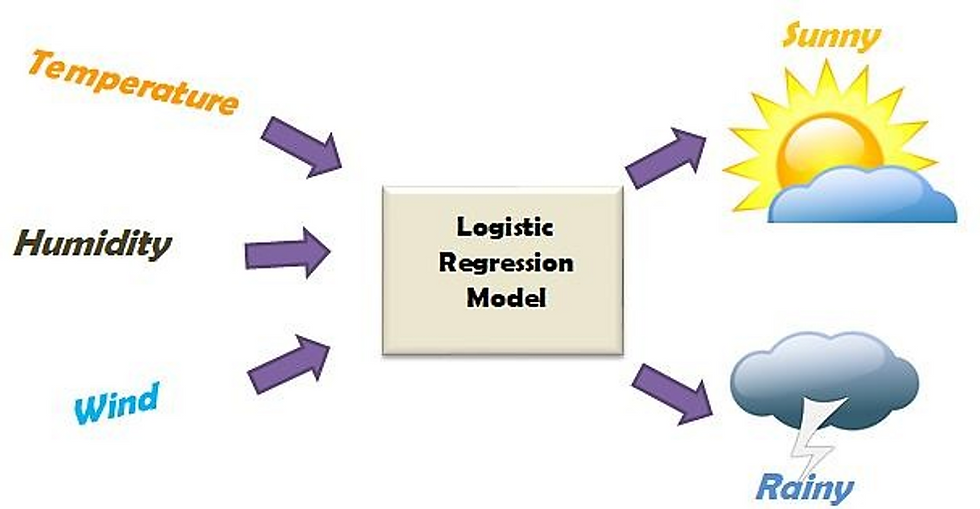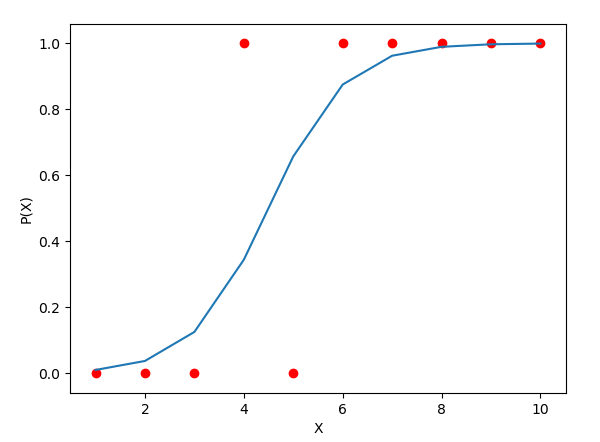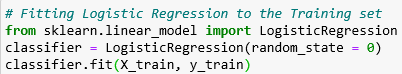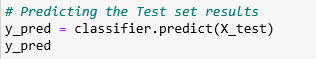top of page

• Sai Manoj

# Machine Learning Algorithm- Logistic Regression## What is Regression?

Regression is one of the most popular supervised learning algorithms in predictive analytics. A regression model requires the knowledge of both the outcome and the feature variables in the dataset.

The following are a few examples of linear regression problems:

1. A hospital may be interested in finding how the total cost of a patient for a treatment varies with the body weight of the patient.

2. Restaurants would like to know the relationship between the customer waiting time after placing the order and the revenue.

3. E-commerce companies such as Amazon, big basket etc would like to understand the impact of variables such as unemployment rate, marital status, balance in the bank account, rainfall etc. on the percentage of non-performing assets(NPA).

4. Insurance companies would like to understand the association between healthcare costs and ageing.

5. An organisation may be interested in finding the relationship between revenue generated from a product and features such as the price, money spent on promotion, competitors price and promotion expenses.

## Supervised Learning

Supervised learning as the name indicates the presence of supervisor as a teacher. The training data will consist of inputs paired with the correct outputs. During training, the algorithm will search for patterns in the data that will correlate with the desired outputs. After training, it will take in new data which will determine which label the new inputs will be classified as based on prior training data. The motive is to predict the correct label for new input data. It can be written as Y=f(x).

## Logistic Regression

It is a Statistical Machine Learning algorithm that classifies the data. It can be applied when the dependent variable is categorical. The goal of logistic regression is to allocate the data to their respective classes based on their relationship. The equation for logistic regression can be written asThe graph formed by logistic regression is given below usually “s” shaped and values at Y-axis will always be between 0 and 1:Examples of logistic regression include:

1. Suppose that we are interested in the factors that influence whether a political candidate wins an election. The outcome (response) variable is binary (0/1); win or lose.The predictor variables of interest are the amount of money spent on the campaign, the amount of time spent campaigning negatively and whether or not the candidate is an incumbent.

3. To find whether an email received is spam or not.

4. To find if a bank loan is granted or not.

5. Whether the tumor is malignant or not.

We have many types of logistic regressions such as -

## 1. Binary Logistic Regression

It is a type of classification with two outcomes. Examples include whether the mail received is spam or not.

## 2. Multinomial Logistic Regression

It is a type of classification with more than two outcomes. Examples include whether the price of a car is expensive, moderate or cheap.

## 3. Ordinal Logistic Regression

It is a type of classification with more outcomes in a sequenced fashion, An example of ordinal logistic regression is rating happiness on a scale of 1–10.

## Import LibrariesNumpy → It is a library used for working with arrays.

Pandas → To load the data file as a Pandas dataframe and analyze the data.

Matplotlib → I’ve imported pyplot to plot graphs of the data.

## Import DatasetOur file is in the CSV(Comma Separated Values) format, so we import the file using pandas. Then we split the data into Dependent and Independent variables. X is considered as Independent and Y is considered as Dependent.

## Train set and Test setFrom Sklearn, sub-library model_selection, I’ve imported the train_test_split which is used to split train and test sets. We can use the train_test_split function to make the split. The 'test_size = 0.25' inside the function indicates the percentage of the data that should be held over for testing.

## Feature Scaling

When we are working with a model it is important to make sure that values are in the same range or else it would be difficult to pass it to the model. To resolve this issue I make us of feature scaling. It is a technique to standardize the independent features present in the data in a fixed range. It is performed during the data pre-processing.

In our dataset, we have four features:

USER ID

AGE

INCOME

OWN HOUSE Here, we treat Age and Income as Independent variables and Own House as a dependent variable. In our independent variables, the age is in terms of ten’s place and Income is in terms of thousands, there is a need to apply feature scaling to these variables to get the best predictions.

The sci-kit learn library provides a class to scale our data, We can use StandaradScaler class from preprocessing. We import the class from the library, we will create an object for it. We will make use of the fit_transform method to transform the train and test set of Independent variables into the same range.## Now let’s fit the data

From Sklearn, a sub-library linear model, we import Linear Regression and we fit the model on the training data.## Predict test results## Evaluation Metrics

Simply building a predictive model is not our motive. It’s about creating and selecting a model that gives high accuracy out of sample data. Hence, it is crucial to check the accuracy of your model before computing predicted values. We will make use of one of the evaluation metrics techniques that are used to calculate the accuracy of classification models. Let’s discuss it in detail.

## Confusion matrix

It is a performance measurement technique for classifications models in Machine Learning where the output has two or more classes. It is a table with four different combinations of actual and predicted values.Let’s understand the terminology in the confusion matrix.

True Positive(TP)

If the actual value and the predicted value are true, then it is a true positive. For example, you predicted that a observation is an apple and observation is an apple.

False Negative(FN)

If the actual value is True and the predicted value is false, then it is a False Negative. For example, you predicted a observation is not an apple but observation is an apple.

False Positive(FP)

If the predicted value is false but the actual value is true, then it is a False Positive. For example, you predicted observation is an apple but observation is not apple.

True Negative(TN)

apple and observation is not an apple.If the predicted value is false and the actual value is also false, then it is a True Negative. For example, you predicted observation is not an apple.Let’s import confusion matrix from metrics class and create an object for it, then we will pass y_test and y_pred as parameters to know the accuracy of predicted values. Here we need to add True Positive and True negative to know the performance of the model.

Here is a summary of what I did: I have loaded in the data, split the data into train and test sets, applied standard scaler method to normalize the data into the same range, fitted a regression model to the training data, made predictions based on this data and tested the predictions on the test data.

Implementation using python: Github

bottom of page